# How to Calculate and Solve for Gas Flow of Reservoir Fluid Flow | The Calculator Encyclopedia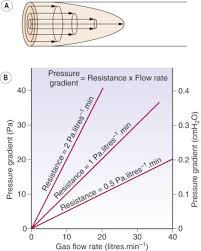The image above represents gas flow.

To compute for the gas flow, four essential parameters are needed and these parameters are Performance Co-efficient (C), Average Reservoir Pressure (Pr*), Flowing Bore Hole Pressure (Pwf*) and Exponent (n).

The formula for calculating the gas flow:

Qg = C(Pr*² – Pwf*²)n

Where;

Qg = Gas Flow
C = Performance Co-efficient
Pr* = Average Reservoir Pressure
Pwf* = Flowing Bore Hole Pressure
n = Exponent

Let’s solve an example;
Find the gas flow when a performance Co-efficient is 9, average reservoir pressure is 10, flowing bore hole pressure is 5 and an exponent of 20 is given.

This implies that;

C = Performance Co-efficient = 9
Pr* = Average Reservoir Pressure = 10
Pwf* = Flowing Bore Hole Pressure = 5
n = Exponent = 20

Qg = C(Pr*² – Pwf*²)n
Qg = 9(10² – 5²)20
Qg = 9(100 – 25)20
Qg = 9(75)20
Qg = 9(3.17e+37)
Qg = 2.85e+38

Therefore, the gas flow is 2.85e+38.

Nickzom Calculator – The Calculator Encyclopedia is capable of calculating the gas flow.

To get the answer and workings of the gas flow using the Nickzom Calculator – The Calculator Encyclopedia. First, you need to obtain the app.

You can get this app via any of these means:

To get access to the professional version via web, you need to register and subscribe for NGN 1,500 per annum to have utter access to all functionalities.
You can also try the demo version via https://www.nickzom.org/calculator

Apple (Paid) – https://itunes.apple.com/us/app/nickzom-calculator/id1331162702?mt=8
Once, you have obtained the calculator encyclopedia app, proceed to the Calculator Map, then click on Petroleum under EngineeringNow, Click on Reservoir Fluid Flow under Petroleum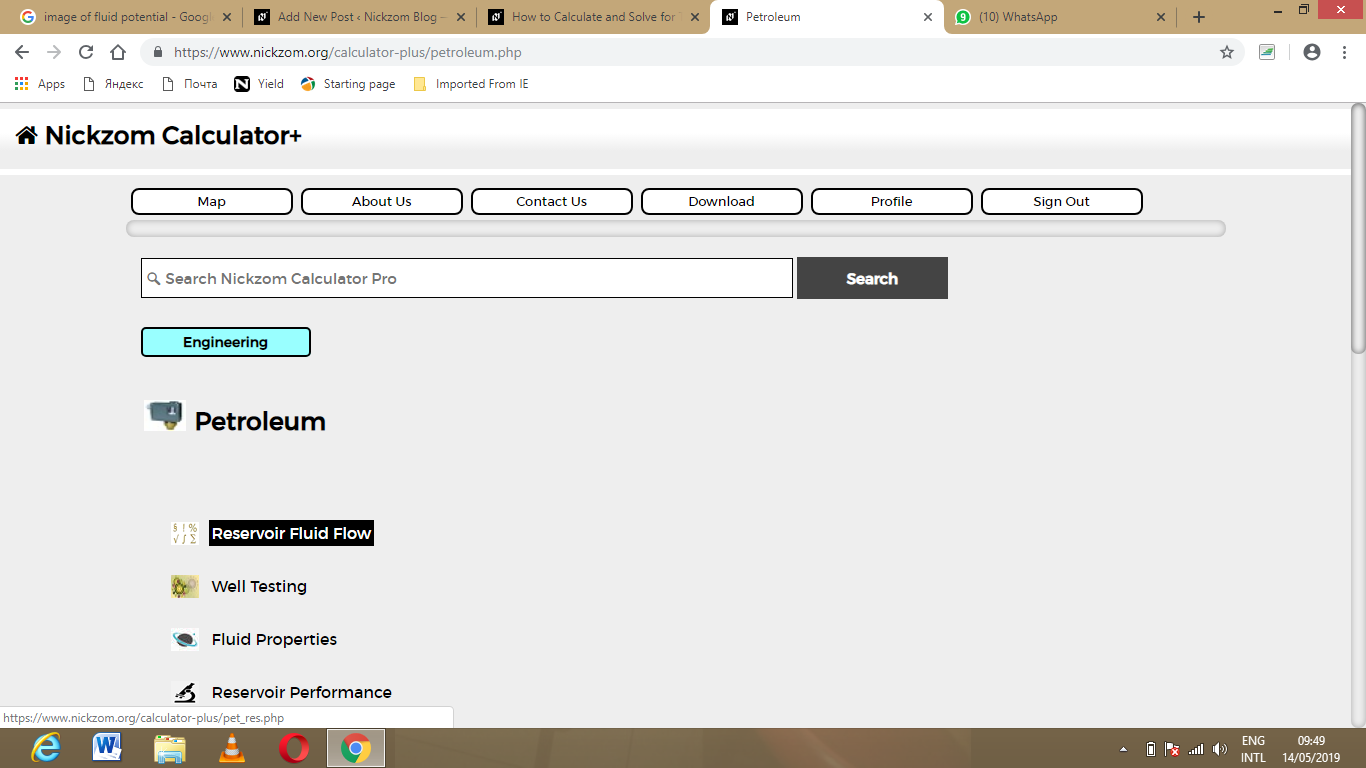Now, Click on Gas Flow under Reservoir Fluid Flow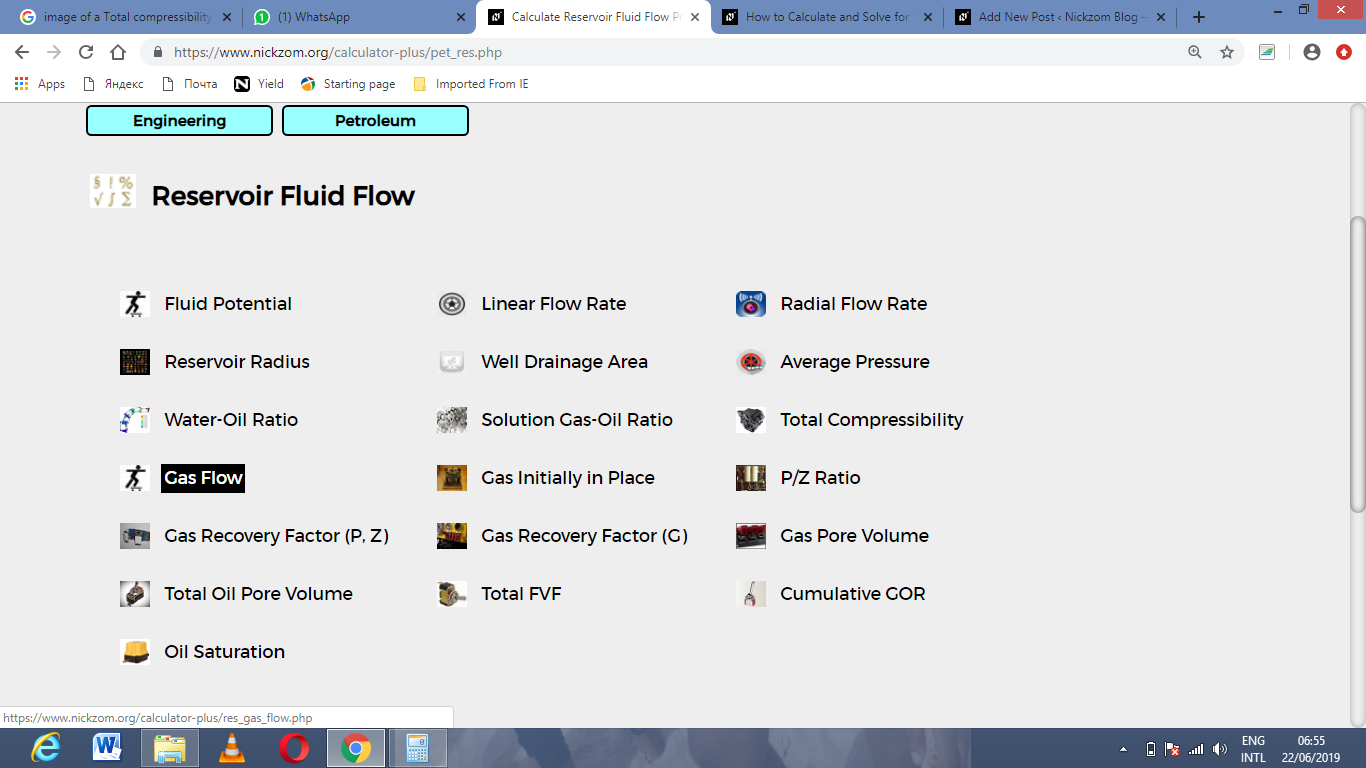The screenshot below displays the page or activity to enter your value, to get the answer for the gas flow according to the respective parameter which are the Performance Co-efficient (C), Average Reservoir Pressure (Pr*), Flowing Bore Hole Pressure (Pwf*) and Exponent (n).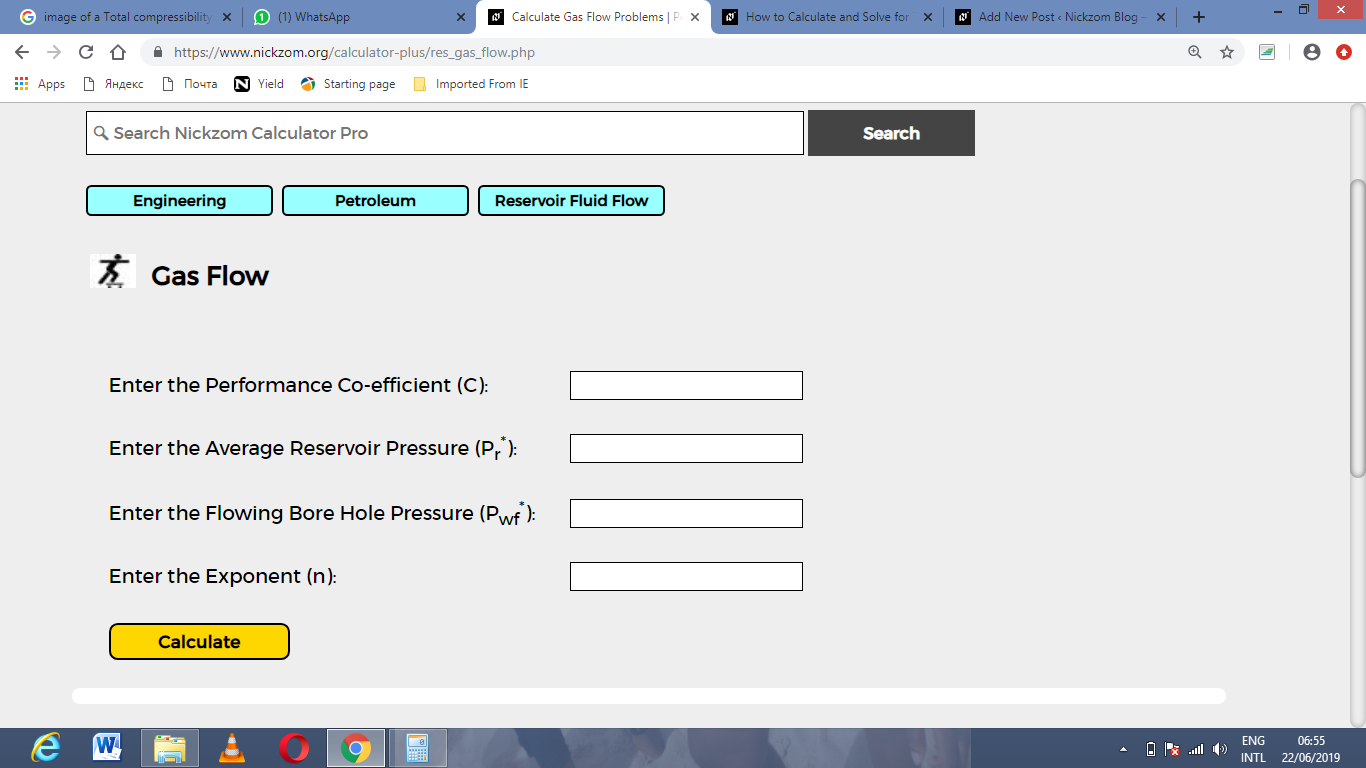Now, enter the value appropriately and accordingly for the parameter as required by the Performance Co-efficient (C) is 9, Average Reservoir Pressure (Pr*) is 10, Flowing Bore Hole Pressure (Pwf*) is 5 and Exponent (n) is 20.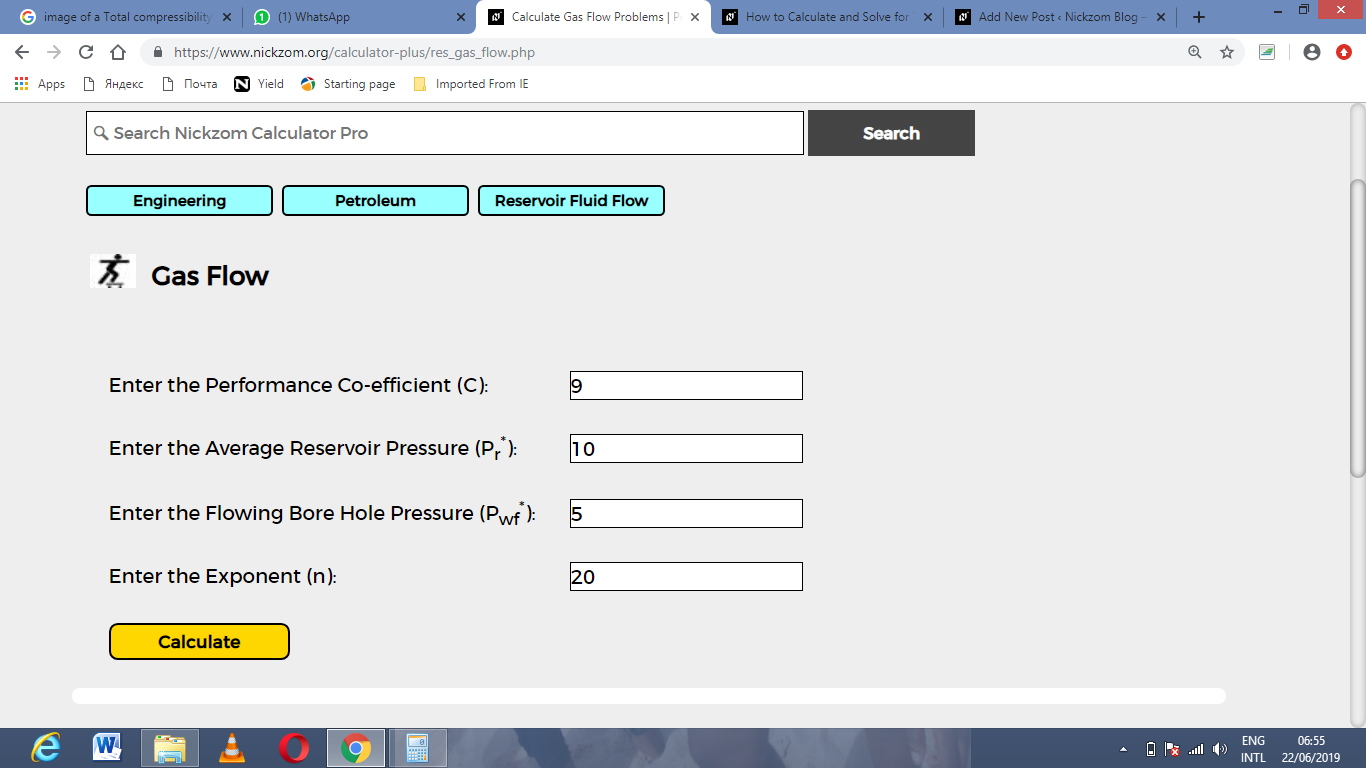Finally, Click on Calculate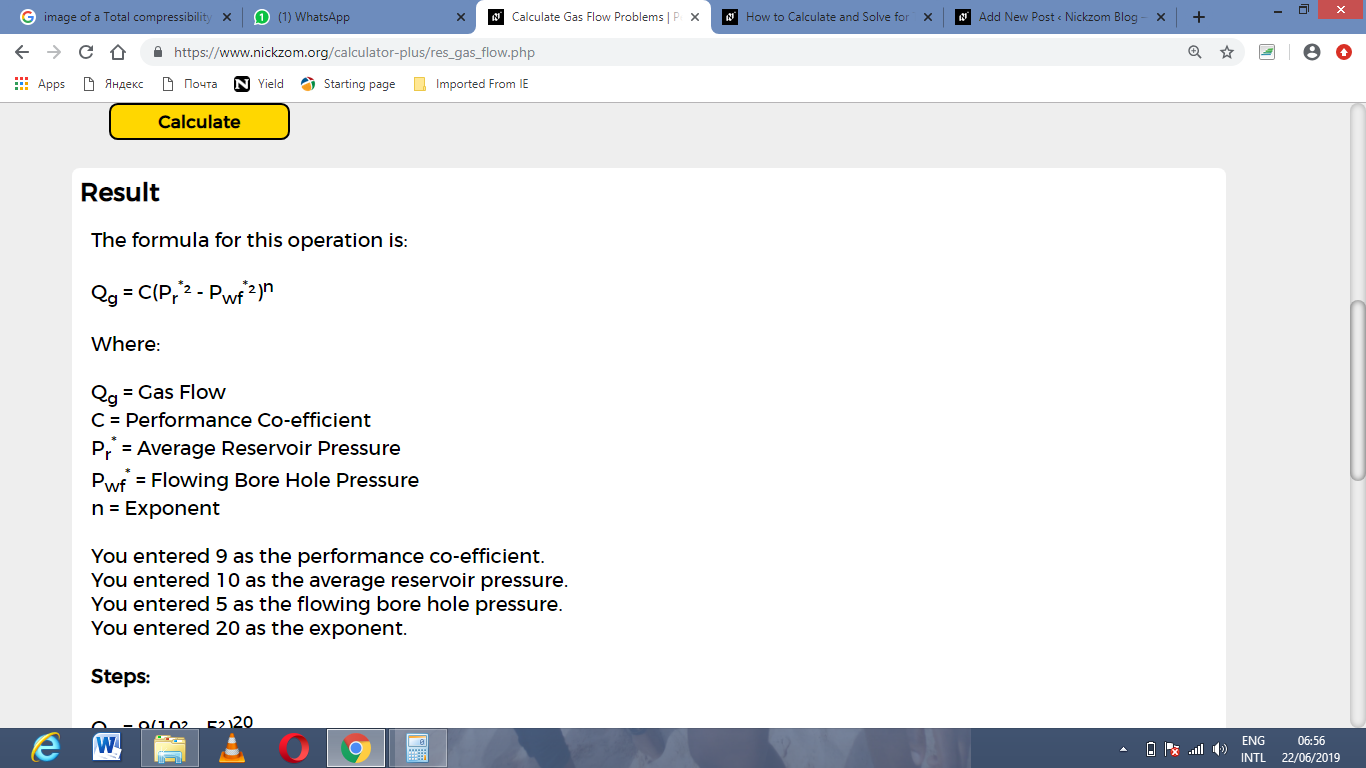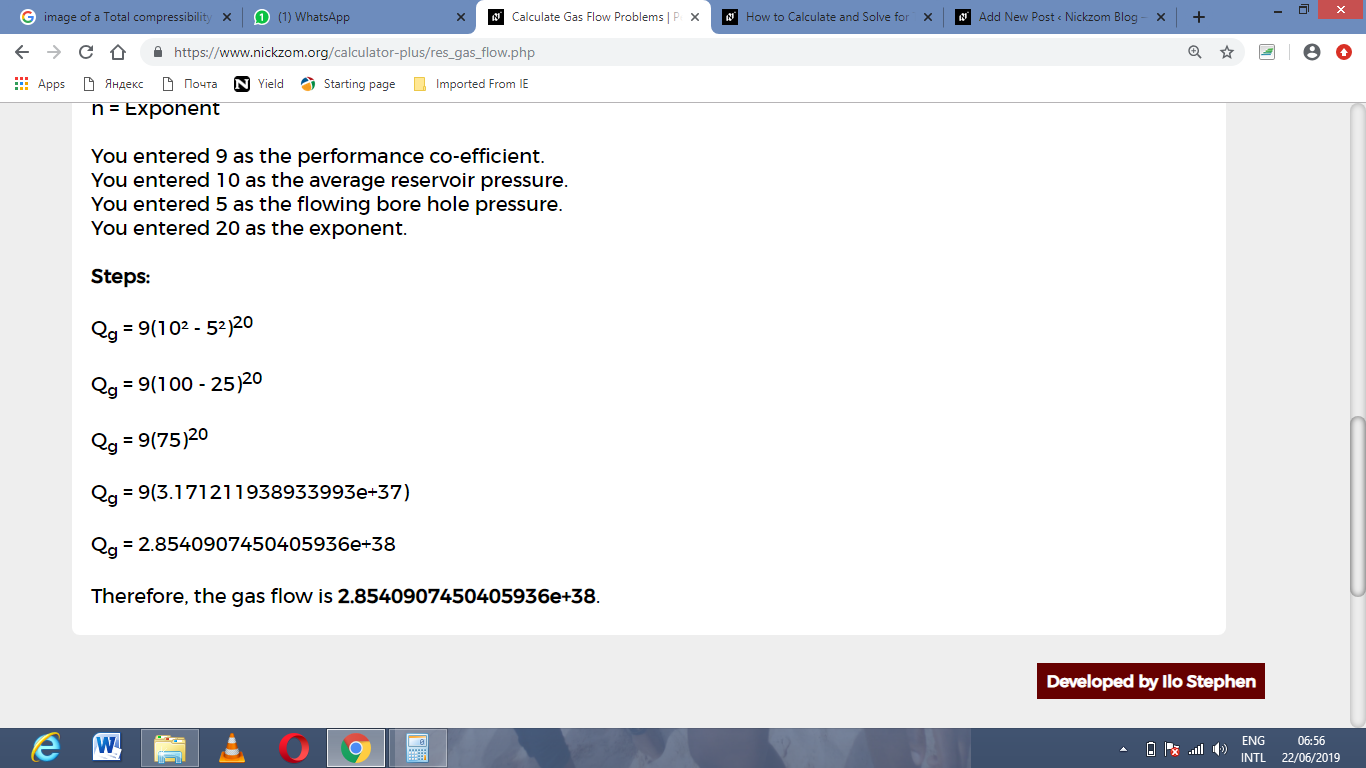As you can see from the screenshot above, Nickzom Calculator– The Calculator Encyclopedia solves for the gas flow and presents the formula, workings and steps too.